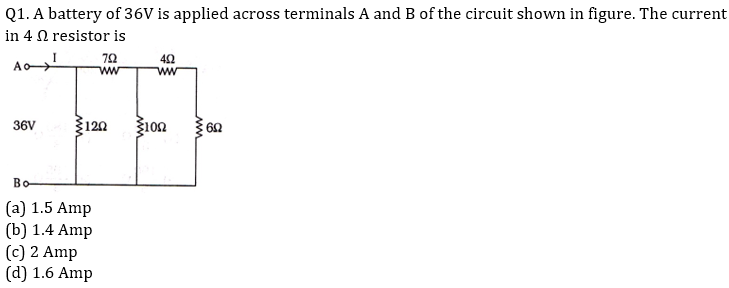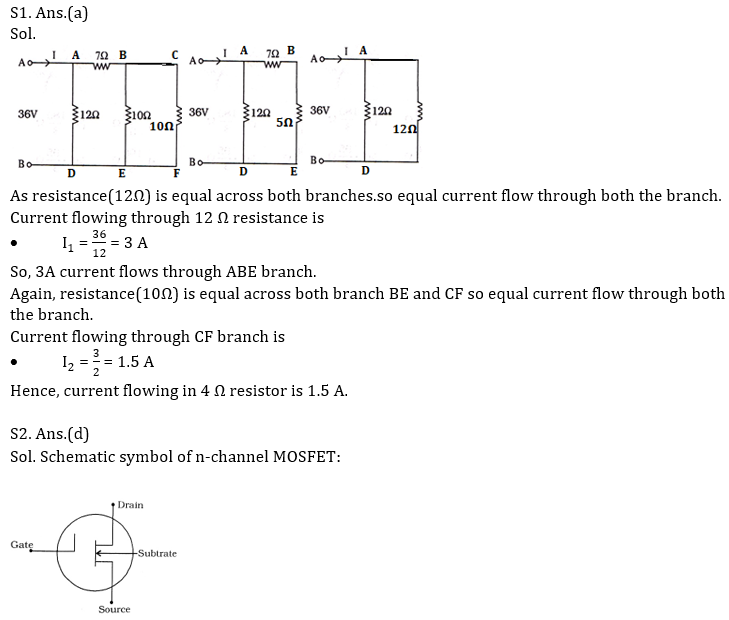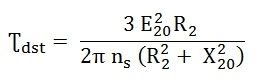Engineering Jobs   »   DFCCIL ELECTRICAL QUIZ

# DFCCIL’21 EE: Daily Practices Quiz 23-July-2021

Each question carries 1 mark.
Negative marking: 1/4 mark
Total Questions: 06
Time: 08 min.Q2. A MOSFET has………terminals.
(a) two
(b) five
(c) four
(d) three

Q3. Strength of the electric field is ___________
(a) Directly proportional to the force applied
(b) Inversely proportional to the force applied
(c) Directly proportional to the square of the force applied
(d) Inversely proportional to the square of the force applied

Q4. Ferranti effect can be compensated by which of the following
(a) Shunt capacitor
(b) Shunt reactor
(c) Series capacitor
(d) Both a and b

Q5. Schering bridge is used to measure
(a) Dielectric loss
(b) The inductance
(c) Low resistance
(d) Mutual Inductance

Q6. If a resistance is inserted in the rotor circuit of a slip ring induction motor, then compared to direct line starting:
(a) Both the starting current and torque reduce
(b) Both the starting current and torque increase
(c) The starting current reduces but starting torque increases
(d) The starting current reduces but starting torque

SOLUTIONSS3. Ans.(a)
Sol. Electric field intensity is the force per unit charge; hence it is directly proportional to the force applied.

S4. Ans.(b)
Sol. Ferranti effect occurs in case of no load or light load condition of a medium and long transmission lines due to capacitance effect of the transmission line.
Shunt reactor will reduce the effect of capacitance. Therefore, Ferranti effect will be reduced.

S5. Ans.(a)
Sol. Schering bridge is used to measure dielectric loss. It is an AC bridge circuit that is used for measuring the insulating properties of electrical cables and equipment. It has the advantage that the balance equation is independent of frequency.
• Maxwell bridge is used to measure the value of unknown inductance.
• Heaviside bridge is used to measured mutual inductance in terms of a known self-inductance.
• Kelvin bridge is used to measure the value of low resistance.

S6. Ans.(c)
Sol. Starting torque is directly proportional to the rotor resistance of an induction motor.In slip ring induction motor the ends of the rotor windings are externally connected by a variable rheostat. So more the resistance, more the torque. When we add resistance to the rotor, the torque is high, the slip is high and the current is reduced. Therefore, slip ring induction motor is used for providing high starting torque.

Sharing is caring!